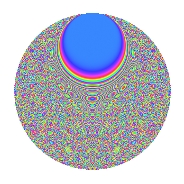# Properties

 Label 2365.2.bwLevel 2365 Weight 2 Character orbit bw Rep. character $$\chi_{2365}(452,\cdot)$$ Character field $$\Q(\zeta_{28})$$ Dimension 2640 Sturm bound 528

# Related objects

## Defining parameters

 Level: $$N$$ = $$2365 = 5 \cdot 11 \cdot 43$$ Weight: $$k$$ = $$2$$ Character orbit: $$[\chi]$$ = 2365.bw (of order $$28$$ and degree $$12$$) Character conductor: $$\operatorname{cond}(\chi)$$ = $$215$$ Character field: $$\Q(\zeta_{28})$$ Sturm bound: $$528$$

## Dimensions

The following table gives the dimensions of various subspaces of $$M_{2}(2365, [\chi])$$.

Total New Old
Modular forms 3216 2640 576
Cusp forms 3120 2640 480
Eisenstein series 96 0 96

## Trace form

 $$2640q - 16q^{6} + O(q^{10})$$ $$2640q - 16q^{6} + 400q^{16} + 32q^{21} - 16q^{23} + 280q^{30} + 64q^{31} - 280q^{32} + 48q^{35} + 2592q^{36} - 24q^{38} + 80q^{40} + 32q^{41} - 104q^{43} + 40q^{47} - 112q^{51} + 8q^{52} - 40q^{53} - 40q^{57} - 280q^{60} + 104q^{68} - 280q^{70} - 280q^{72} - 112q^{73} - 168q^{78} + 240q^{81} - 140q^{82} - 56q^{83} - 296q^{86} - 392q^{87} + 8q^{90} - 104q^{92} - 120q^{95} + 256q^{96} + O(q^{100})$$

## Decomposition of $$S_{2}^{\mathrm{new}}(2365, [\chi])$$ into newform subspaces

The newforms in this space have not yet been added to the LMFDB.

## Decomposition of $$S_{2}^{\mathrm{old}}(2365, [\chi])$$ into lower level spaces

$$S_{2}^{\mathrm{old}}(2365, [\chi]) \cong$$ $$S_{2}^{\mathrm{new}}(215, [\chi])$$$$^{\oplus 2}$$

## Hecke Characteristic Polynomials

There are no characteristic polynomials of Hecke operators in the database